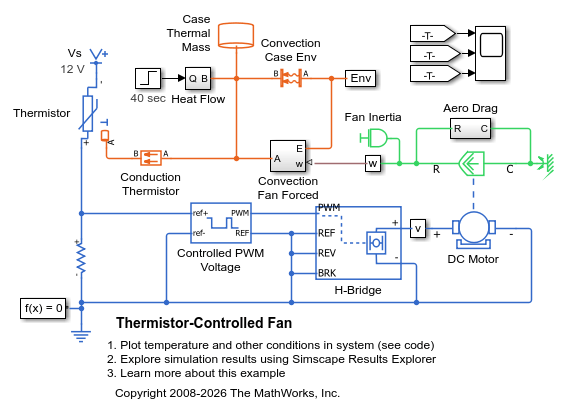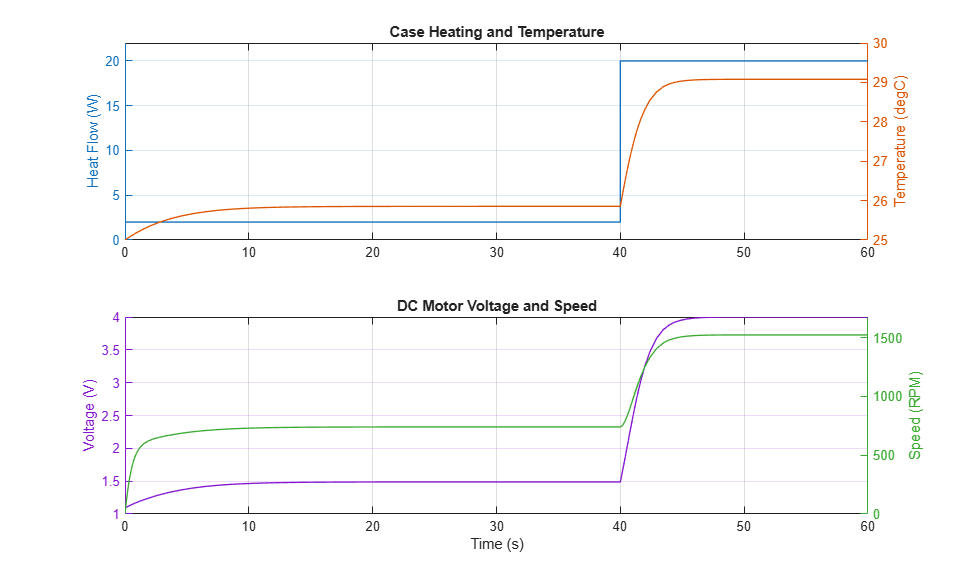# Thermistor-Controlled Fan

This model shows how fundamental thermal, mechanical and electrical components can be used to model a thermistor-controlled fan. The heat-generating device starts producing 2 watts at time zero, and then at 40 seconds this increases to 20 watts. The thermistor therefore heats up, and its resistance decreases thereby increasing the voltage across the PWM reference pins. This increases the PWM frequency which in turn increases average motor current, and the fan speeds up. The additional fan speed increases the convective cooling of the device, moderating the temperature increase of the device.

This is a system-level model such as might be used for selecting an appropriate thermistor characteristic. The convective heat transfer coefficient used to model nominal cooling (i.e. when the fan isn't running) would typically be determined by experiment. Knowing the temperature difference and having an estimate of the device area, the heat transfer coefficient can be calculated. The coefficient for the fan-assisted cooling could then be estimated by running the motor at maximum RPM, and again measuring the temperature difference. The nominal cooling term just also be taken into account when calculating the fan-cooling coefficient.

The Controlled PWM Voltage and H-Bridge blocks have two modes of operation, namely Averaged and PWM. As this is a system-level model, and the thermal time constants are measured in seconds, the Averaged mode of operation is used. The PWM mode replicates the PWM control signal which would typically operate at a few kilohertz.

### Model### Simulation Results from Simscape Logging

The plot below shows the electrical, mechanical, and thermal behavior of the thermistor controlled motor. As the temperature of the thermistor changes, the voltage applied to the motor changes, which alters the speed of the motor and the convective heat transfer from the case. The system reaches steady state after a short period of time.### Results from Real-Time Simulation

This example has been tested on a Speedgoat Performance real-time target machine with an Intel® 3.5 GHz i7 multi-core CPU. This model can run in real time with a step size of 300 microseconds.